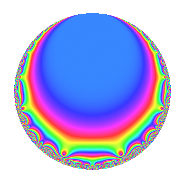# Properties

 Label 65.2.a.cLevel 65 Weight 2 Character orbit 65.a Self dual Yes Analytic conductor 0.519 Analytic rank 0 Dimension 2 CM No Inner twists 1

# Related objects

## Newspace parameters

 Level: $$N$$ = $$65 = 5 \cdot 13$$ Weight: $$k$$ = $$2$$ Character orbit: $$[\chi]$$ = 65.a (trivial)

## Newform invariants

 Self dual: Yes Analytic conductor: $$0.519027613138$$ Analytic rank: $$0$$ Dimension: $$2$$ Coefficient field: $$\Q(\sqrt{3})$$ Coefficient ring: $$\Z[a_1, a_2]$$ Coefficient ring index: $$1$$ Fricke sign: $$-1$$ Sato-Tate group: $\mathrm{SU}(2)$

## $q$-expansion

Coefficients of the $$q$$-expansion are expressed in terms of $$\beta = \sqrt{3}$$. We also show the integral $$q$$-expansion of the trace form.

 $$f(q)$$ $$=$$ $$q + \beta q^{2} + ( 1 - \beta ) q^{3} + q^{4} - q^{5} + ( -3 + \beta ) q^{6} + 2 q^{7} -\beta q^{8} + ( 1 - 2 \beta ) q^{9} +O(q^{10})$$ $$q + \beta q^{2} + ( 1 - \beta ) q^{3} + q^{4} - q^{5} + ( -3 + \beta ) q^{6} + 2 q^{7} -\beta q^{8} + ( 1 - 2 \beta ) q^{9} -\beta q^{10} + ( -3 + \beta ) q^{11} + ( 1 - \beta ) q^{12} + q^{13} + 2 \beta q^{14} + ( -1 + \beta ) q^{15} -5 q^{16} + 2 \beta q^{17} + ( -6 + \beta ) q^{18} + ( -1 + 3 \beta ) q^{19} - q^{20} + ( 2 - 2 \beta ) q^{21} + ( 3 - 3 \beta ) q^{22} + ( 3 + \beta ) q^{23} + ( 3 - \beta ) q^{24} + q^{25} + \beta q^{26} + 4 q^{27} + 2 q^{28} + ( -6 - 2 \beta ) q^{29} + ( 3 - \beta ) q^{30} + ( 5 - 3 \beta ) q^{31} -3 \beta q^{32} + ( -6 + 4 \beta ) q^{33} + 6 q^{34} -2 q^{35} + ( 1 - 2 \beta ) q^{36} -4 q^{37} + ( 9 - \beta ) q^{38} + ( 1 - \beta ) q^{39} + \beta q^{40} -2 \beta q^{41} + ( -6 + 2 \beta ) q^{42} + ( 5 + 3 \beta ) q^{43} + ( -3 + \beta ) q^{44} + ( -1 + 2 \beta ) q^{45} + ( 3 + 3 \beta ) q^{46} + 6 q^{47} + ( -5 + 5 \beta ) q^{48} -3 q^{49} + \beta q^{50} + ( -6 + 2 \beta ) q^{51} + q^{52} -6 \beta q^{53} + 4 \beta q^{54} + ( 3 - \beta ) q^{55} -2 \beta q^{56} + ( -10 + 4 \beta ) q^{57} + ( -6 - 6 \beta ) q^{58} + ( -3 - 7 \beta ) q^{59} + ( -1 + \beta ) q^{60} + ( 2 + 6 \beta ) q^{61} + ( -9 + 5 \beta ) q^{62} + ( 2 - 4 \beta ) q^{63} + q^{64} - q^{65} + ( 12 - 6 \beta ) q^{66} + ( -4 - 6 \beta ) q^{67} + 2 \beta q^{68} -2 \beta q^{69} -2 \beta q^{70} + ( 3 - \beta ) q^{71} + ( 6 - \beta ) q^{72} -4 q^{73} -4 \beta q^{74} + ( 1 - \beta ) q^{75} + ( -1 + 3 \beta ) q^{76} + ( -6 + 2 \beta ) q^{77} + ( -3 + \beta ) q^{78} + ( 2 + 6 \beta ) q^{79} + 5 q^{80} + ( 1 + 2 \beta ) q^{81} -6 q^{82} -6 q^{83} + ( 2 - 2 \beta ) q^{84} -2 \beta q^{85} + ( 9 + 5 \beta ) q^{86} + 4 \beta q^{87} + ( -3 + 3 \beta ) q^{88} + ( -6 + 4 \beta ) q^{89} + ( 6 - \beta ) q^{90} + 2 q^{91} + ( 3 + \beta ) q^{92} + ( 14 - 8 \beta ) q^{93} + 6 \beta q^{94} + ( 1 - 3 \beta ) q^{95} + ( 9 - 3 \beta ) q^{96} + 2 q^{97} -3 \beta q^{98} + ( -9 + 7 \beta ) q^{99} +O(q^{100})$$ $$\operatorname{Tr}(f)(q)$$ $$=$$ $$2q + 2q^{3} + 2q^{4} - 2q^{5} - 6q^{6} + 4q^{7} + 2q^{9} + O(q^{10})$$ $$2q + 2q^{3} + 2q^{4} - 2q^{5} - 6q^{6} + 4q^{7} + 2q^{9} - 6q^{11} + 2q^{12} + 2q^{13} - 2q^{15} - 10q^{16} - 12q^{18} - 2q^{19} - 2q^{20} + 4q^{21} + 6q^{22} + 6q^{23} + 6q^{24} + 2q^{25} + 8q^{27} + 4q^{28} - 12q^{29} + 6q^{30} + 10q^{31} - 12q^{33} + 12q^{34} - 4q^{35} + 2q^{36} - 8q^{37} + 18q^{38} + 2q^{39} - 12q^{42} + 10q^{43} - 6q^{44} - 2q^{45} + 6q^{46} + 12q^{47} - 10q^{48} - 6q^{49} - 12q^{51} + 2q^{52} + 6q^{55} - 20q^{57} - 12q^{58} - 6q^{59} - 2q^{60} + 4q^{61} - 18q^{62} + 4q^{63} + 2q^{64} - 2q^{65} + 24q^{66} - 8q^{67} + 6q^{71} + 12q^{72} - 8q^{73} + 2q^{75} - 2q^{76} - 12q^{77} - 6q^{78} + 4q^{79} + 10q^{80} + 2q^{81} - 12q^{82} - 12q^{83} + 4q^{84} + 18q^{86} - 6q^{88} - 12q^{89} + 12q^{90} + 4q^{91} + 6q^{92} + 28q^{93} + 2q^{95} + 18q^{96} + 4q^{97} - 18q^{99} + O(q^{100})$$

## Embeddings

For each embedding $$\iota_m$$ of the coefficient field, the values $$\iota_m(a_n)$$ are shown below.

For more information on an embedded modular form you can click on its label.

Label $$\iota_m(\nu)$$ $$a_{2}$$ $$a_{3}$$ $$a_{4}$$ $$a_{5}$$ $$a_{6}$$ $$a_{7}$$ $$a_{8}$$ $$a_{9}$$ $$a_{10}$$
1.1
 −1.73205 1.73205
−1.73205 2.73205 1.00000 −1.00000 −4.73205 2.00000 1.73205 4.46410 1.73205
1.2 1.73205 −0.732051 1.00000 −1.00000 −1.26795 2.00000 −1.73205 −2.46410 −1.73205
 $$n$$: e.g. 2-40 or 990-1000 Significant digits: Format: Complex embeddings Normalized embeddings Satake parameters Satake angles

## Inner twists

This newform does not admit any (nontrivial) inner twists.

## Atkin-Lehner signs

$$p$$ Sign
$$5$$ $$1$$
$$13$$ $$-1$$

## Hecke kernels

This newform can be constructed as the kernel of the linear operator $$T_{2}^{2} - 3$$ acting on $$S_{2}^{\mathrm{new}}(\Gamma_0(65))$$.